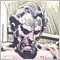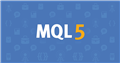# When to use buffers55

I have been studying the buffers section of the documentation. It appears that they are only used to draw lines (or maybe text also) to the screen.

Is this a correct assumption?201053142

CaptainPablo:

I have been studying the buffers section of the documentation. It appears that they are only used to draw lines (or maybe text also) to the screen.

Is this a correct assumption?

I think of Indicator buffers as:

"Un-dimensioned arrays of type double managed by the indicator."

Basically, buffers hold the actual calculated data values, which are generally calculated by some formula you supply. The general purpose of an indicator is to converts price data into some different format for some different way of visualizing the data.55

Thanks for the input Anthony and William. And I have been doing some more studying. So it looks like the buffers are primarily for plotting the data from an indicator. But I don't need to use buffers if I want to draw a horizontal line at some price for example.

It is sinking in slowly. Thanks for you help. I have a lot more reading and re-reading to do.

I don't need a response. I'll save it for when I am really stuck.

Thinking of the indicator data as an array is helpful. I know I saw that somewhere but it just sunk in.Documentation on MQL5: Constants, Enumerations and Structures / Objects Constants / Object Types
• www.mql5.com
When a graphical object is created using the ObjectCreate() function, it's necessary to specify the type of object being created, which can be one of the values of the ENUM_OBJECT enumeration. Further specifications of object properties are possible using functions for working with graphical objects.618

CaptainPablo:

So it looks like the buffers are primarily for plotting the data from an indicator.

Not only for plotting.

Think of buffers as a sequence of the results and preliminary results of your indicator's calculation steps. Some of them you might want to plot, others you just need to store for future calculations.

Let's take a simple Bollinger Bands indicator as an example: you're plotting 3 lines: a central moving average band plus an upper and lower band within a distance of n standard deviations. In other words: you don't plot the standard deviation itself, but you need it as a preliminary calculation in order to get the values for your upper and lower band, so reserving one of the buffers for the standard deviation is one possibility - even if it's not plotted.

As a more general scenario, we often need to use results of previous iterations (=calls of the OnCalculate function) again, because with indicators we often deal with calculating things like the mean (moving average), standard deviations, ranges, momentum ...etc. within a "sliding window" that slides along the time axis. With every new step only the last element of such a "sliding window" is new, all others were already used for previous iterations, so it would be a waste of computation power to re-calculate them over and over again, so the "buffers" are just the number sequences holding such values for easy access.38391

Chris70:

Not only for plotting.

Think of buffers as a sequence of the results and preliminary results of your indicator's calculation steps. Some of them you might want to plot, others you just need to store for future calculations.

Let's take a simple Bollinger Bands indicator as an example: you're plotting 3 lines: a central moving average band plus an upper and lower band within a distance of n standard deviations. In other words: you don't plot the standard deviation itself, but you need it as a preliminary calculation in order to get the values for your upper and lower band, so reserving one of the buffers for the standard deviation is one possibility - even if it's not plotted.

As a more general scenario, we often need to use results of previous iterations (=calls of the OnCalculate function) again, because with indicators we often deal with calculating things like the mean (moving average), standard deviations, ranges, momentum ...etc. within a "sliding window" that slides along the time axis. With every new step only the last element of such a "sliding window" is new, all others were already used for previous iterations, so it would be a waste of computation power to re-calculate them over and over again, so the "buffers" are just the number sequences holding such values for easy access.

Right. And not only for internal calculations.

There is also an other possible use for buffers, it's to exchange information/data from an indicator to an other code (EA/Script/Indicator or even a Service). It's however a 1 way communication channel (you can't provide updated data back to the indicator. It can be very useful in some complex situations.55

Chris70:

Not only for plotting.

Think of buffers as a sequence of the results and preliminary results of your indicator's calculation steps. Some of them you might want to plot, others you just need to store for future calculations.

Let's take a simple Bollinger Bands indicator as an example: you're plotting 3 lines: a central moving average band plus an upper and lower band within a distance of n standard deviations. In other words: you don't plot the standard deviation itself, but you need it as a preliminary calculation in order to get the values for your upper and lower band, so reserving one of the buffers for the standard deviation is one possibility - even if it's not plotted.

As a more general scenario, we often need to use results of previous iterations (=calls of the OnCalculate function) again, because with indicators we often deal with calculating things like the mean (moving average), standard deviations, ranges, momentum ...etc. within a "sliding window" that slides along the time axis. With every new step only the last element of such a "sliding window" is new, all others were already used for previous iterations, so it would be a waste of computation power to re-calculate them over and over again, so the "buffers" are just the number sequences holding such values for easy access.

I see. So the buffers are the way to access the data in the indicator, to plot or to access the info for other calculations.55

Alain Verleyen:

Right. And not only for internal calculations.....

Thank you. I think I am getting my head wrapped around this.38391

CaptainPablo:

Thank you. I think I am getting my head wrapped around this.

If you are beginner yo don't need to worry about this last point I posted. I did it for completeness, but you will certainly not need that to start.